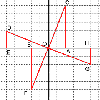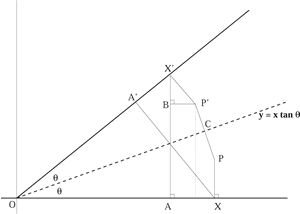#### You may also like### 8 Methods for Three by One

This problem in geometry has been solved in no less than EIGHT ways by a pair of students. How would you solve it? How many of their solutions can you follow? How are they the same or different? Which do you like best?### Reflect Again

Follow hints to investigate the matrix which gives a reflection of the plane in the line y=tanx. Show that the combination of two reflections in intersecting lines is a rotation.### The Matrix

Investigate the transfomations of the plane given by the 2 by 2 matrices with entries taking all combinations of values 0. -1 and +1.

# Rots and Refs

##### Age 16 to 18 Challenge Level:

The point $P$ has coordinates $(r\cos \phi, r\sin \phi)$ where the distance of $P$ from the origin is $OP=r$ and the line $OP$ is at angle $\phi$ to the x axis. Find the image of this point under the transformation given by the matrix

$\mathbf{T_1}=\left( \begin{array}{cc} cos \theta & -\sin \theta\\ sin \theta & cos \theta \end{array} \right)$

Draw a diagram and describe the effect of this transformation on the points of the plane.

The point $P$ has coordinates $(p,q)$ and the point $P'$ is the reflection of $P$ in the line $y = x\tan\theta$. In the diagram below the lines $P'X'$ and $XA'$ are perpendicular to the line $OA'X'$. "Prove that $OX = OX' = p$, $P'X' = PX = q$ and $OA = OA' = p\cos 2\theta.$ Find the lengths $BP',\ AX'$ and $BX'$ and hence prove that transformation given by the matrix

$\mathbf{T_2}=\left( \begin{array}{cc} cos 2\theta & sin2\theta\\ sin2\theta & -\cos2\theta \end{array} \right)$

gives a reflection in the line $y=\tan\theta$.